# 基于复化Simpson公式改进的灰色神经网络组合模型对国民总收入的预测The Grey Neural Network Combination Model Based on Complex Simpson Formula is Used to Predict the Gross National Income of China

DOI: 10.12677/SA.2018.76068, PDF, HTML, XML, 下载: 502  浏览: 839  科研立项经费支持

Abstract: Aiming at the non-linearity and randomness of China's gross national income data, this paper establishes a grey neural network combined forecasting model which is improved by using the complex Simpson formula to predict China's gross national income. Firstly, the GM(1,1) model and the GM(1,1) model based on the complex Simpson integral formula to improve the background value are established. Secondly, a BP neural network prediction model is established. Furthermore, the GM(1,1) model and the BP neural network prediction model improved by the complex Simpson formula are optimally linearly weighted, and the objective function is solved by using the genetic algorithm to obtain the objective function with the smallest squared error sum. Weighting factors are to improve prediction accuracy. Finally, the GM(1,1) model, the GM(1,1) model of the modified Simpson formula, the BP neural network model and the improved gray neural network model of the modified Simpson formula are used to compare the results of the gross national income forecast. From the prediction results, the gray neural network model improved by the complex Simpson formula is significantly better than the GM(1,1) model, the GM(1,1) model of the modified Simpson formula and the BP neural network model.

1. 引言

GNI，即国民总收入(Gross National Income)，是指“一国经济总体当期从国内生产和国外生产中获得的生产性收入总量”，是该经济体所有常住单位在一定时期内收入初次分配的最终结果。GNI等于GDP加上来自国外的净要素收入，一般来说来自国外的要素净收入远小于本国GDP的规模，因此GNI与GDP往往规模相当，二者都被用于反映一国的经济规模，见(蒋萍，2011  ；侯瑜，2012  )。因此，科学、准确地对人均GNI做出预测为政府部门有效的调控经济政策和制定发展规划有着重要的作用。

2. 研究方法

2.1. GM(1,1)预测模型

${x}^{\left(1\right)}\left(k\right)=\underset{i=1}{\overset{k}{\sum }}{x}^{\left(0\right)}\left(i\right)\left(k=1,2,\cdots ,n\right)$ (1)

${Z}^{\left(1\right)}$${X}^{\left(1\right)}$ 的紧邻均值生成序列： ${Z}^{\left(1\right)}=\left({z}^{\left(1\right)}\left(1\right),{z}^{\left(1\right)}\left(2\right),\cdots ,{z}^{\left(1\right)}\left(n\right)\right)$ ，其中：

${z}^{\left(1\right)}\left(k\right)=0.5\left({x}^{\left(1\right)}\left(k\right)+{x}^{\left(1\right)}\left(k-1\right)\right),\text{\hspace{0.17em}}\text{\hspace{0.17em}}k=2,3,\cdots ,n$ (2)

${x}^{\left(0\right)}\left(k\right)+a{z}^{\left(1\right)}\left(k\right)=b,k=2,3,\cdots ,n$ (3)

$B=\left[\begin{array}{cc}-{z}^{\left(1\right)}\left(2\right)& 1\\ -{z}^{\left(1\right)}\left(3\right)& 1\\ ⋮& ⋮\\ -{z}^{\left(1\right)}\left(n\right)& 1\end{array}\right],Y=\left[\begin{array}{c}{x}^{\left(0\right)}\left(2\right)\\ {x}^{\left(0\right)}\left(3\right)\\ ⋮\\ {x}^{\left(0\right)}\left(n\right)\end{array}\right]$ (4)

$\stackrel{^}{a}={\left({B}^{\text{T}}B\right)}^{-1}{B}^{\text{T}}Y$ (5)

$B,Y,\stackrel{^}{u}$ 满足式(4)和式(5)，相应的白化微分方程为

$\frac{\text{d}{x}^{\left(1\right)}}{\text{d}t}+a{x}^{\left(1\right)}=b$ (6)

${\stackrel{^}{x}}^{\left(1\right)}\left(k+1\right)=\left({x}^{\left(0\right)}\left(1\right)-\frac{b}{a}\right){\text{e}}^{-ak}+\frac{b}{a},\text{\hspace{0.17em}}\text{\hspace{0.17em}}k=1,2,\cdots ,n$ (7)

$\begin{array}{c}{\stackrel{^}{x}}^{\left(0\right)}\left(k+1\right)={\stackrel{^}{x}}^{\left(1\right)}\left(k+1\right)-{\stackrel{^}{x}}^{\left(1\right)}\left(k\right)\\ =\left(1-{\text{e}}^{a}\right)\left({x}^{\left(0\right)}\left(1\right)-\frac{b}{a}\right){\text{e}}^{-ak},\text{\hspace{0.17em}}\text{\hspace{0.17em}}k=1,2,\cdots ,n\end{array}$ (8)

2.2. 背景值误差产生分析

b，的是 ${z}^{\left(1\right)}\left(k\right)$ ，因此GM(1,1)模型误差主要来源于 ${z}^{\left(1\right)}\left(k\right)$ 的计算。对 $\frac{\text{d}{x}^{\left(1\right)}\left(t\right)}{\text{d}t}+a{x}^{\left(1\right)}\left(t\right)=b$$\left[k-1,k\right]$ 进行积分，有：

$I={\int }_{k-1}^{k}\text{d}{x}^{\left(1\right)}\left(t\right)+a{\int }_{k-1}^{k}{x}^{\left(1\right)}\left(t\right)\text{d}t=b{\int }_{k-1}^{k}\text{d}t,\text{\hspace{0.17em}}\text{\hspace{0.17em}}k=2,3,\cdots ,n-1$ (9)

${z}^{\left(1\right)}\left(k\right)={\int }_{k-1}^{k+1}{x}^{\left(1\right)}\left(t\right)\text{d}t$ (10)

GM(1,1)模型的背景值的几何意义如图所示，可以明显看出，曲线 ${x}^{\left(1\right)}\left(t\right)$ 在区间 $\left[k-1,k\right]$ 上与横轴t所围成的曲边梯形的面积即是GM(1,1)模型的背景值。传统计算 ${z}^{\left(1\right)}\left(k\right)$ 的方式是计算梯形的面积，如图1所示。而用复化Simpson公式计算的GM(1,1)模型，背景值是区间 $\left[k-1,k\right]$ 上的曲边梯形的面积，如图2所示。可以看出，其计算产生的误差明显小于传统的计算方式。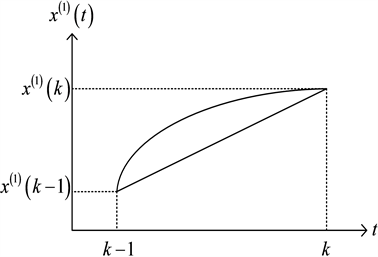Figure 1. Comparison between the real value and the trapezoid formula background value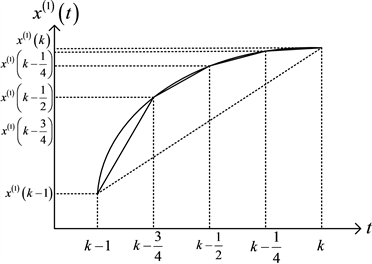Figure 2. Comparison of the true value and the background value of complex Simpson formula

2.3. 基于复化Simpson公式改进的GM(1,1)模型

$\alpha =\mathrm{ln}\frac{{x}^{\left(0\right)}\left(k\right)}{{x}^{\left(0\right)}\left(k-1\right)}$ (11)

$A=\frac{{x}^{\left(0\right)}\left(k\right)}{{\left(\frac{{x}^{\left(0\right)}\left(k\right)}{{x}^{\left(0\right)}\left(k-1\right)}\right)}^{k-1}-\left(1-\frac{{x}^{\left(0\right)}\left(k-1\right)}{{x}^{\left(0\right)}\left(k\right)}\right)}$ (12)

$B={x}^{\left(0\right)}\left({k}_{1}\right)-A={x}^{\left(0\right)}\left({k}_{1}\right)-\frac{{x}^{\left(0\right)}\left(k\right)}{{\left(\frac{{x}^{\left(0\right)}\left(k\right)}{{x}^{\left(0\right)}\left(k-1\right)}\right)}^{k-1}-\left(1-\frac{{x}^{\left(0\right)}\left(k-1\right)}{{x}^{\left(0\right)}\left(k\right)}\right)}$ (13)

${x}^{\left(1\right)}\left(t\right)=A{\text{e}}^{\alpha \left(t-1\right)}+B,\text{\hspace{0.17em}}t\ge 1$ 为其1-AGO动态序列预测模型。

${x}_{{i}_{k-1}}^{\left(1\right)}={x}^{\left(1\right)}\left(k-1+{i}_{k-1}h\right),\left(i=0,1,2,\cdots ,2m\right),\text{\hspace{0.17em}}\text{\hspace{0.17em}}h=\frac{1}{p},\text{\hspace{0.17em}}m=\frac{p}{2},\text{\hspace{0.17em}}m=1,2,\cdots ,\frac{p}{2}$ (14)

$I={\int }_{k-1}^{k}\text{d}{x}^{\left(1\right)}\left(t\right)+a{\int }_{k-1}^{k}{x}^{\left(1\right)}\left(t\right)\text{d}t=b{\int }_{k-1}^{k}\text{d}t,\text{\hspace{0.17em}}\text{\hspace{0.17em}}k=2,3,\cdots ,n$ (15)

$I={x}^{\left(1\right)}\left(k\right)-{x}^{\left(1\right)}\left(k-1\right)+a{\int }_{k-1}^{k}{x}^{\left(1\right)}\left(t\right)\text{d}t=b,\text{\hspace{0.17em}}\text{\hspace{0.17em}}k=2,3,\cdots ,n$ (16)

$\begin{array}{c}{I}_{1}={\int }_{k-1}^{k}{x}^{\left(1\right)}\left(t\right)\text{d}t\\ =\frac{1}{3}\left[{x}^{\left(1\right)}\left(k\right)-{x}^{\left(1\right)}\left(k-1\right)+2\underset{{i}_{k-1}}{\overset{m-1}{\sum }}{x}_{2{i}_{k-1}}+4\underset{{i}_{k-1}}{\overset{m}{\sum }}{x}_{2{i}_{k-1}-1}\right],\text{\hspace{0.17em}}\text{\hspace{0.17em}}k=2,3,\cdots ,n\end{array}$ (17)

$\begin{array}{c}I={x}^{\left(1\right)}\left(k\right)-{x}^{\left(1\right)}\left(k-1\right)+a{\int }_{k-1}^{k}{x}^{\left(1\right)}\left(t\right)\text{d}t\\ ={x}^{\left(0\right)}\left(k\right)+\frac{a}{3}\left[{x}^{\left(1\right)}\left(k\right)-{x}^{\left(1\right)}\left(k-1\right)+2\underset{{i}_{k-1}}{\overset{m-1}{\sum }}{x}_{2{i}_{k-1}}+4\underset{{i}_{k-1}}{\overset{m}{\sum }}{x}_{2{i}_{k-1}-1}\right]\\ =b,\text{\hspace{0.17em}}\text{\hspace{0.17em}}k=2,3,\cdots ,n\end{array}$ (18)

${z}^{\left(1\right)}\left(k\right)=\frac{a}{3}\left[{x}^{\left(1\right)}\left(k\right)-{x}^{\left(1\right)}\left(k-1\right)+2\underset{{i}_{k-1}}{\overset{m-1}{\sum }}{x}_{2{i}_{k-1}}+4\underset{{i}_{k-1}}{\overset{m}{\sum }}{x}_{2{i}_{k-1}-1}\right],\text{\hspace{0.17em}}\text{\hspace{0.17em}}k=2,3,\cdots ,n$ (19)

$3{x}^{\left(0\right)}\left(k\right)+a\left[{x}^{\left(1\right)}\left(k\right)-{x}^{\left(1\right)}\left(k-1\right)+2\underset{{i}_{k-1}}{\overset{m-1}{\sum }}{x}_{2{i}_{k-1}}+4\underset{{i}_{k-1}}{\overset{m}{\sum }}{x}_{2{i}_{k-1}-1}\right]-3b=0,\text{\hspace{0.17em}}\text{\hspace{0.17em}}k=2,3,\cdots ,n$ (20)

$u={\left[a,b\right]}^{\text{T}}$ 为参数列，由最小二乘法原理知，参数列需满足：

$\stackrel{^}{u}={\left({B}_{2}^{\text{T}}{B}_{2}\right)}^{-1}{B}_{2}^{\text{T}}{Y}_{2}$ (21)

${B}_{2}=\left(\begin{array}{cc}-\frac{1}{3}\left[{x}^{\left(1\right)}\left(2\right)+{x}^{\left(1\right)}\left(1\right)+2\underset{{i}_{1}}{\overset{m-1}{\sum }}{x}_{2{i}_{1}}+4\underset{{i}_{1}}{\overset{m}{\sum }}{x}_{2{i}_{1}-1}\right]& 1\\ -\frac{1}{3}\left[{x}^{\left(1\right)}\left(3\right)+{x}^{\left(1\right)}\left(2\right)+2\underset{{i}_{2}}{\overset{m-1}{\sum }}{x}_{2{i}_{2}}+4\underset{{i}_{2}}{\overset{m}{\sum }}{x}_{2{i}_{2}-1}\right]& 1\\ ⋮& ⋮\\ -\frac{1}{3}\left[{x}^{\left(1\right)}\left(n-1\right)+{x}^{\left(1\right)}\left(n-2\right)+2\underset{{i}_{n-2}}{\overset{m-1}{\sum }}{x}_{2{i}_{n-2}}+4\underset{{i}_{n-2}}{\overset{m}{\sum }}{x}_{2{i}_{n-2}-1}\right]& 1\\ -\frac{1}{3}\left[{x}^{\left(1\right)}\left(n\right)+{x}^{\left(1\right)}\left(n-1\right)+2\underset{{i}_{n-1}}{\overset{m-1}{\sum }}{x}_{2{i}_{n-1}}+4\underset{{i}_{n-1}}{\overset{m}{\sum }}{x}_{2{i}_{n-1}-1}\right]& 1\end{array}\right)$ , ${Y}_{2}=\left(\begin{array}{c}{x}^{\left(0\right)}\left(2\right)\\ {x}^{\left(0\right)}\left(3\right)\\ ⋮\\ {x}^{\left(0\right)}\left(n-1\right)\\ {x}^{\left(0\right)}\left(n\right)\end{array}\right)$ (22)

2.4. BP神经网络

BP (back-propagation)神经网络是由Rumelhard等科学家于20世纪80年代提出的，BP神经网络是一种按误差反向传播算法训练的多层前馈网络，也是目前应用最广泛的神经网络模型之一。BP神经网络具有很强的非线性映射能力，并且网络的隐含层数、各层的处理单元数及网络的学习系数等参数可根据问题的具体情况而设定，具有很高的灵活性，因此被应用到很多领域。图3展示了BP神经网络的简单结构拓扑图：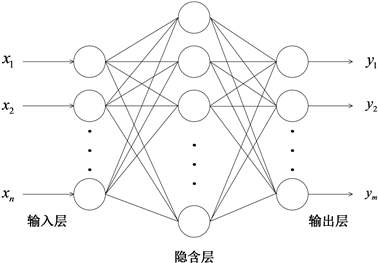Figure 3. BP network structure topology diagram

2.4.1. 神经元模型

$Y=f\left(wp+b\right)$ (23)

2.4.2. 激励函数

BP神经网络的功能的不同主要取决于激励函数的不同，激励函数选择的多样性使得神经网络有了不同的信息处理能力。神经元输入层与输出层之间的关系由激励函数f决定。神经网络的激励函数有多种，本文使用的激励函数为S型函数，其表达式为：

$f\left(x\right)=\frac{1}{1+{\text{e}}^{-cx}}$ (24)

2.4.3. BP神经网络的学习算法

${W}_{1}={\left({w}_{ij}^{1}\right)}_{l×m},\text{\hspace{0.17em}}\text{\hspace{0.17em}}i=1,2,\cdots ,l;\text{\hspace{0.17em}}j=1,2,\cdots ,m$ (25)

${W}_{2}={\left({w}_{ij}^{2}\right)}_{n×l},\text{\hspace{0.17em}}\text{\hspace{0.17em}}i=1,2,\cdots ,n;\text{\hspace{0.17em}}j=1,2,\cdots ,l$ (26)

${O}_{j}=f\left(\underset{i=1}{\overset{m}{\sum }}{w}_{ji}^{2}{x}_{i}-{\theta }_{l}^{1}\right)=f\left(ne{t}_{j}\right),\text{\hspace{0.17em}}\text{\hspace{0.17em}}j=1,2,\cdots ,l$ (27)

$ne{t}_{j}=\underset{i=1}{\overset{m}{\sum }}{w}_{ji}{x}_{i}-{\theta }_{l}^{1}$ (28)

$f\left(·\right)$ 为隐含层传递函数。

${z}_{k}=g\left(\underset{j=1}{\overset{l}{\sum }}{w}_{kj}^{2}{O}_{j}-{\theta }_{k}^{2}\right)=g\left(ne{t}_{k}\right),\text{\hspace{0.17em}}\text{\hspace{0.17em}}k=1,2,\cdots ,n$ (29)

$ne{t}_{k}=\underset{j=1}{\overset{l}{\sum }}{w}_{kj}{O}_{j}-{\theta }_{k}^{2},\text{\hspace{0.17em}}\text{\hspace{0.17em}}k=1,2,\cdots ,n$ (30)

$g\left(·\right)$ 为输出层传递函数。

$E=\frac{1}{2}\underset{k=1}{\overset{n}{\sum }}{\left(yk-{z}_{k}\right)}^{2}$ (31)

$\Delta {w}_{ji}^{1}=-\eta \frac{\partial E}{\partial {w}_{ji}^{1}}$ (32)

$\Delta {w}_{ji}^{2}=-\eta \frac{\partial E}{\partial {w}_{kj}^{2}}$ (33)

2.5. 复化Simpson神经网络组合预测模型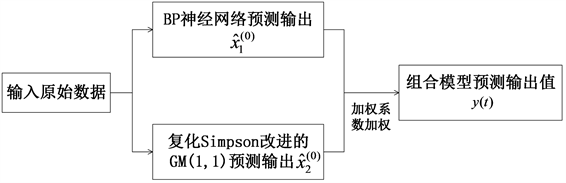Figure 4. BP network structure topology diagram

${\stackrel{^}{x}}_{1}^{\left(0\right)}\left(t\right)$${\stackrel{^}{x}}_{2}^{\left(0\right)}\left(t\right)$$\stackrel{^}{y}\left(t\right)$ 分别表示BP神经网络模型、复化Simpson公式改进的GM(1,1)模型和组合预测模型在t时刻的预测输出值， ${w}_{1}$${w}_{2}$ 为BP神经网络模型、复化Simpson公式改进的GM(1,1)模型的组合权系数，则：

$\stackrel{^}{y}\left(t\right)={w}_{1}{\stackrel{^}{x}}_{1}^{\left(0\right)}\left(t\right)+{w}_{2}{\stackrel{^}{x}}_{2}^{\left(0\right)}\left(t\right)$ (34)

${w}_{1}$${w}_{2}$ 满足

$\left\{\begin{array}{l}{w}_{1}+{w}_{2}=1\\ 0\le {w}_{1},{w}_{2}\le 1\end{array}$ (35)

${e}_{1}\left(t\right)$${e}_{2}\left(t\right)$$e\left(t\right)$ 分别为BP神经网络模型、CSGM(1,1)模型、组合预测模型在t时刻的误差，则：

$\left\{\begin{array}{l}{\stackrel{^}{x}}_{1}^{\left(0\right)}\left(t\right)-{x}^{\left(0\right)}\left(t\right)={e}_{1}\left(t\right)\\ {\stackrel{^}{x}}_{2}^{\left(0\right)}\left(t\right)-{x}^{\left(0\right)}\left(t\right)={e}_{2}\left(t\right)\\ {w}_{1}{\stackrel{^}{x}}_{1}^{\left(0\right)}\left(t\right)+{w}_{2}{\stackrel{^}{x}}_{2}^{\left(0\right)}\left(t\right)=e\left(t\right)\end{array}$ (36)

$e=\underset{t=1}{\overset{n}{\sum }}{|e\left(t\right)|}^{2}$ ，即误差绝对值平方和，进一步表示为：

$\mathrm{min}e=\underset{t=1}{\overset{n}{\sum }}{|e\left(t\right)|}^{2}=\underset{t=1}{\overset{n}{\sum }}{|{w}_{1}{e}_{1}\left(t\right)+{w}_{2}{e}_{2}\left(t\right)|}^{2}$ (37)

$\left\{\begin{array}{l}\frac{\partial e}{\partial {w}_{1}}=\underset{t=1}{\overset{n}{\sum }}2\left({w}_{1}{e}_{1}\left(t\right)+{w}_{2}{e}_{2}\left(t\right)\right){e}_{1}\left(t\right)=0\\ \frac{\partial e}{\partial {w}_{2}}=\underset{t=1}{\overset{n}{\sum }}2\left({w}_{1}{e}_{1}\left(t\right)+{w}_{2}{e}_{2}\left(t\right)\right){e}_{2}\left(t\right)=0\end{array}$ (38)

$E=\left[\begin{array}{cccc}{e}_{1}\left(1\right)& {e}_{1}\left(2\right)& \cdots & {e}_{1}\left(n\right)\\ {e}_{2}\left(1\right)& {e}_{2}\left(2\right)& \cdots & {e}_{2}\left(n\right)\end{array}\right]$ (39)

$W=\left[\begin{array}{c}{w}_{1}{e}_{1}\left(1\right)+{w}_{2}{e}_{2}\left(1\right)\\ {w}_{1}{e}_{1}\left(2\right)+{w}_{2}{e}_{2}\left(2\right)\\ ⋮\\ {w}_{1}{e}_{1}\left(n\right)+{w}_{2}{e}_{2}\left(n\right)\end{array}\right]$ (40)

$EW=0$ (41)

3. 我国国民总收入预测Table 1. Actual GNI data from 2008 to 2016

4. 预测结果Table 2. Comparison of the predicted and actual GNI values of China from 2008 to 2016 based on different models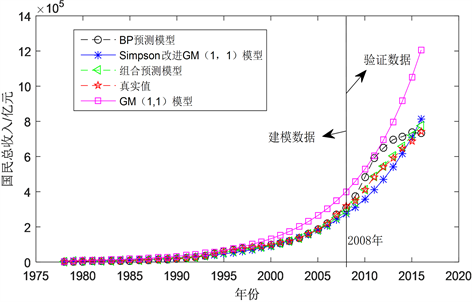Figure 5. Comparison of predicted and actual GNI values of China from 1978 to 2016 based on different modelsTable 3. Relative error between predicted and actual GNI of per capita based on different models

5. 结论Figure 6. GNI relative error comparison graphs based on different models in verified data

1) 复化Simpson公式改进的灰色神经网络组合模型用于中国国民总收入(GNI)的方法是切实有效的。

2) 复化Simpson公式改进的灰色神经网络组合模型预测的精度最高，RMSPEPO为4.4687%。其预测精度高于GM(1,1)模型、BP神经网络模型和复化Simpson改进的GM(1,1)模型。

3) 组合预测的预测精度比单个预测精度更高。同时，它可以克服单一模型预测的欠缺，综合结合各种预测模型的长处，能够更好地降低预测的误差。因此，组合预测模型可以达到改观单一预测结果的目的。进一步期望本文模型能应用于人口、能源等领域。

  蒋萍. 核算制度缺陷, 统计方法偏颇与经济总量失实[M]. 北京: 中国统计出版社.  侯瑜. 基于DQAF框架的我国统计数据质量管理及改进[J]. 统计研究, 2012, 29(12): 24-30.  侯青霞. 中国人均GDP的函数系数自回归模型的预测研究[D]: [硕士学位论文]. 西安: 西安理工大学, 2008.  张雅清. 基于残差修正的GM(1,1)模型在山西省人均GDP预测中的应用[J]. 攀枝花学院学报, 2016, 33(2): 68-70.  王永杰. 人工神经网络算法在GDP和CPI中的预测应用[D]: [硕士学位论文]. 太原: 中北大学, 2017.  沈艳, 张丽玲. 基于复合辛普森公式的GM(1,1)模型背景值的优化[J]. 应用科技, 2016, 43(4): 81-84.  蒋诗泉, 刘思峰, 周兴才. 基于复化梯形公式的GM(1,1)模型背景值的优化[J]. 控制与决策, 2014, 29(12): 2221-2225.